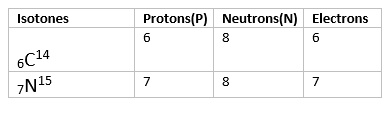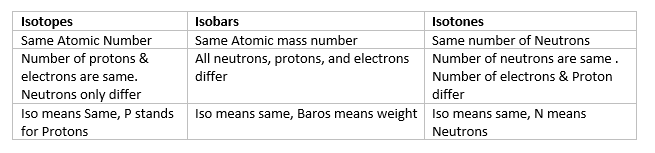# Isotones

## Isotones

• Species having same number of neutrons but different number of protons are called Isotones.
• Example $^{14}_{6}C$, $^{15}_{7}N$• Other examples includes boron-12 and carbon-13 nuclei both contain 7 neutrons, and so are isotones. Similarly, $^{36}S$, $^{37}Cl$, $^{38}Ar$, $^{39}K$, and $^{40}Ca$ nuclei are all isotones of 20 because they all contain 20 neutrons.
• the term was formed by the German physicist K. Guggenheimer by changing the "p" in "isotope" from "p" for "proton" to "n" for "neutron".

## Difference Between Isotopes,Isobars & Isotones## Solved Examples

Question 1
$^{40}_{20}X$ and $^{40}_{21}X$ are
(i) Isobars
(ii) Isotopes
(iii) Isotones
(iv) Isostereomers
Solution
(i)

Question 2
Isotones are elements having
(i) Same mass number but different neutrons
(ii) Same atomic number but different neutrons
(iii) Same atomic number, mass number and neutrons
(iv) Different atomic number and atomic mass number but same neutrons
Solution
(iv)

Question 3
Which of the following species is isotonic with $^{86}_{37}X$
(i) $^{84}_{36}Y$
(ii) $^{85}_{37}Y$
(iii) $^{89}_{41}Y$
(iv) $^{87}_{38}Y$
Solution
(iv)

Question 4
Which of the following is an isotonic pair
(i) $^{40}_{19}K$,$^{40}_{20}Ca$
(ii)$^{40}_{18}Ar$,$^{40}_{20}Ca$
(iii) $^{33}_{18}Ar$,$^{40}_{18}Ar$
(iv)$^{39}_{19}K$,$^{40}_{20}Ca$
Solution
(iv)